# Factorial ring

(diff) ← Older revision | Latest revision (diff) | Newer revision → (diff)

A ring with unique decomposition into factors. More precisely, a factorial ringis an integral domain in which one can find a system of irreducible elementssuch that every non-zero element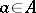admits a unique representation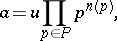whereis invertible and the non-negative integral exponents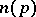are non-zero for only a finite number of elements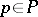. Here an element is called irreducible inif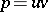implies that eitheroris invertible in, andis not invertible in.

In a factorial ring there is a highest common divisor and a least common multiple of any two elements. A ringis factorial if and only if it is a Krull ring and satisfies one of the following equivalent conditions: 1) every divisorial ideal ofis principal; 2) every prime ideal of height 1 is principal; and 3) every non-empty family of principal ideals has a maximal element, and the intersection of any two principal ideals is principal. Every principal ideal ring is factorial. A Dedekind ring is factorial only if it is a principal ideal ring. Ifis a multiplicative system in a factorial ring, then the ring of fractions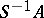is factorial. A Zariski ringis factorial if its completionis.

Subrings and quotient rings of a factorial ring need not be factorial. The ring of polynomials over a factorial ring and the ring of formal power series over a field or over a discretely-normed ring are factorial. But the ring of formal power series over a factorial ring need not be factorial.

An integral domain is factorial if and only if its multiplicative semi-group is Gaussian (see Gauss semi-group), and for this reason factorial rings are also called Gaussian rings or Gauss rings.

How to Cite This Entry:
Factorial ring. Encyclopedia of Mathematics. URL: http://encyclopediaofmath.org/index.php?title=Factorial_ring&oldid=15956
This article was adapted from an original article by L.V. Kuz'min (originator), which appeared in Encyclopedia of Mathematics - ISBN 1402006098. See original article